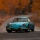Home
IT Knowledge
Inspiration
Languages
EN

# Java - how to round a number to two decimal places?

0 pointsVadim-Kotiv
294

Hi!

How to round the result so that there are always two places after the decimal point, e.g. 56.99? I tried to use `Math.round()` but unfortunately it doesn't work :(

0 pointsVadim-Kotiv
294

After a little research here is the solution I found:

Rounding float numbers is not super easy, and it takes some time to understand. It's best to go over a lot of examples and write a few utils.

In general, the issue of floats is quite a broad topic in programming and computer science. For beginners, I suggest not going into too much detail, as it may be a bit discouraged by the amount of information available on this topic.

## Example 1 with Math.round - multiplication and division

Here we have a rounding method and some examples of its use. I suggest to copy this example and modify it to better understand the action.

``````public class RoundExample1 {

public static void main(String[] args) {

System.out.println(roundTo2DecimalPlace(56.990));  // 56.99
System.out.println(roundTo2DecimalPlace(56.994));  // 56.99
System.out.println(roundTo2DecimalPlace(56.995));  // 57.0
System.out.println(roundTo2DecimalPlace(56.996));  // 57.0
System.out.println(roundTo2DecimalPlace(56.999));  // 57.0
}

public static double roundTo2DecimalPlace(double value) {
return Math.round(value * 100.0) / 100.0;
}
}``````

## Example 2 with BigDecimal

The precision is set to the second place after the opposite, so for the third example, 56.995 we get: 56.99 instead of 57.0

``````import java.math.BigDecimal;
import java.math.RoundingMode;

public class RoundExample2 {

public static void main(String[] args) {

System.out.println(round(56.990)); // 56.99
System.out.println(round(56.991)); // 56.99
System.out.println(round(56.995)); // 56.99
System.out.println(round(56.996)); // 57.0
System.out.println(round(56.999)); // 57.0
}

public static double round(double value) {
int precision = 2;
BigDecimal bigDecimal = new BigDecimal(value);
bigDecimal = bigDecimal.setScale(precision, RoundingMode.HALF_UP);
return bigDecimal.doubleValue();
}
}``````